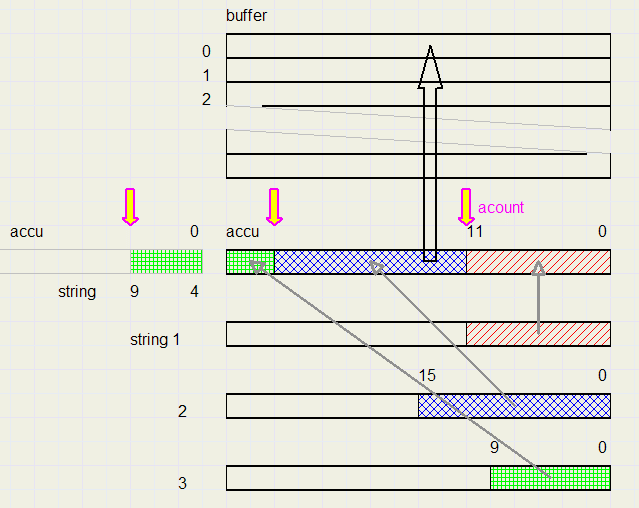Delphi programming trics: handling serial bit streamsIntroduction

This problem was part of a project for image compression..

Operations

Bit strings of varying length are presented to be stored in a buffer.
Also, bit streams of varying length are to be extracted from a buffer.
The buffer is an array of dwords (cardinal).
The bit packages are first assembled into a 32 bit register, called ACCU.
When the accu is full, it is copied to the buffer and a pointer is updated to address the next entry in the buffer.
Below, the data flow is illustrated.Acount counts the number of bits present in the Accu.
When accu reaches a count of 32 (or more) it has to be saved into the buffer.
In the picture above, the accu is shown twice for clarity.

Below is the procedure for the storing of bit streams.
The buffer is named "director" , it's index is dirPTR.
A dword containing n bits must be stored (n < 32)
```procedure storeCode(w : dword; n : byte);
//store n bits of w to dirtab (via accu)
//n = 1 ..16
var space : byte;
begin
space := 32 - acount;
accu := accu or (w shl acount);
if space >= n then            //if space
begin
acount := acount + n;
if acount = 32 then
begin
acount := 0;
director[dirPtr] := accu;
inc(dirPtr);
accu := 0;
end;
end
else                         //if no space
begin
director[dirPtr] := accu;
accu := 0;
inc(dirPtr);
accu := w shr space;
acount := n - space;
end;
end;
```
When a new bitstring is presented, there are two possibilities:

1. The accu has space
acount is increased by n
w is left shifted to the accu by acount positions
if acount = 32, then it must be written to the buffer

2. The accu has no space
The (partial) bitsteam is left shifted into the accu, just as in case 1.
Then the bitstream is right shifted into the accu by the amount of the original space.
Acount is set to the bitstream length - the space.

For the extraction of bitstreams from the buffer a similar approach holds.
Below is the function that extracts (reads) n bits:
```function readcode(n : byte) : dword;
//read n bits from director table
space : byte;
begin
if acount= 0 then
begin
accu := director[dirPtr];
inc(dirPtr);
end;
mask := (1 shl n) -1;
space := 32 - acount;
result := accu shr acount;
acount := acount + n;
if acount = 32 then acount := 0
else if acount > 32 then
begin
accu := director[dirPtr];
inc(dirPtr);
dec(acount,32);
result := (result or (accu shl space));
end;
end;
```

Termination

When terminating, there may be unstored bits in the accu.
To store them issue
storecode(0, 32-acount);

Global data

The following data must be declared outside the read and write bitstream procedures:
```var director    : array[0..maxdir] of dword;
dirPtr      : dword;
accu        : dword;  //bit asembly register
acount      : byte;   //nr of valid bitsin accu
```
Before starting the storage or reading of strings, variables dirPtr, accu, acount must be set to zero.